# Using Lookup and Reference Functions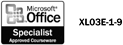You can use lookup and reference functions in Excel to make it easy to retrieve information from a data list. The lookup functions (VLOOKUP and HLOOKUP) allow you to search for and insert a value in a cell that is stored in another place in the worksheet. The HLOOKUP function looks in rows (a horizontal lookup) and the VLOOKUP function looks in columns (a vertical lookup). Each function uses four arguments (pieces of data) as shown in the following definition: =VLOOKUP (lookup_value, table_array, col_index_num, range_lookup). The VLOOKUP function finds a value in the left-most column of a named range and returns the value from the specified cell to the right of the cell with the found value, while the HLOOKUP function does the same to rows. In the example, =VLOOKUP(12,Salary,2,TRUE), the function looks for the value 12 in the named range Salary and finds the closest ( next lower) value, and returns the value in column 2 of the same row and places the value in the active cell. In the example, =HLOOKUP ("Years",Salary,4,FALSE), the function looks for the value "Years" in the named range Salary and finds the exact text string value, and then returns the value in row 4 of the column.

#### Use the VLOOKUP Function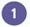Create a data range in which the left-most column contains a unique value in each row.Click the cell you want to place the function.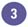Type =VLOOKUP( value, named range, column, TRUE or FALSE), and then press Enter.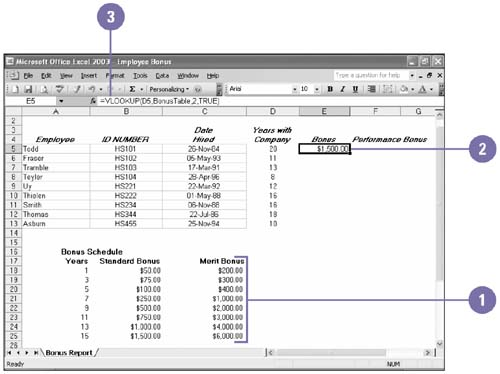#### Use the HLOOKUP FunctionCreate a data range in which the uppermost row contains a unique value in each row.Click the cell you want to place the function.Type =HLOOKUP( value, named range, row, TRUE or FALSE) , and then press Enter.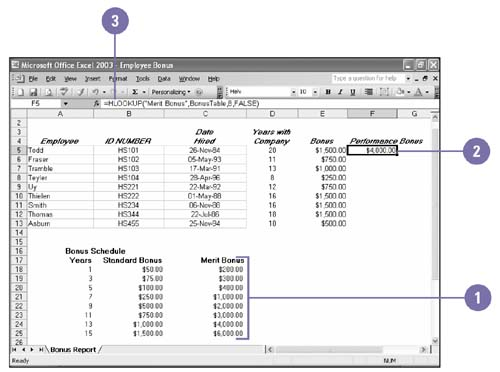## Lookup Function Arguments

Argument

Description

lookup_value

The value found in the row or the column of the named range. You can use a value, cell reference or a text string ( enclosed in quotation marks).

table_array

The named range of information in which Excel looks up data.

col_index_num

The numeric position of the column in the named range (counting from the left) for the value to be returned (use only for VLOOKUP).

row_index_num

The numeric position of the row in the named range (counting from the top) for the value to be returned (use only for HLOOKUP).

range_lookup

The value returned when the function is to find the nearest value (TRUE) or an exact match (FALSE) for the lookup_value. The default value is TRUE.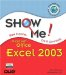Show Me Microsoft Office Excel 2003
ISBN: 0789730057
EAN: 2147483647
Year: 2002
Pages: 291